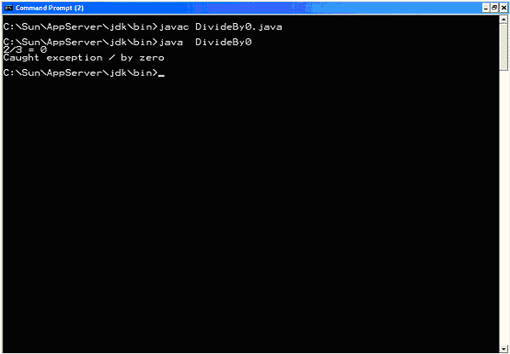## Core Java

Java Evolution
Overview of Java Language
Constant, Variable and Datatype
Operator and Expression
Decision making, Branching and Looping
Class Object and Method
Array and String
Inheritance
Package
 Here application creates a method and forces it to divide by zero. The method does not have to explicitly throw an exception because the division operator throws an exception when required. An example of a built-in exception. import java.io.* ; import java.lang.Exception ; public class DivideBy0 { public static void main( String[] args ) { int a = 2 ; int b = 3 ; int c = 5 ; int d = 0 ; int e = 1 ; int f = 3 ; try { System.out.println( a+"/"+b+" = "+div( a, b ) ) ; System.out.println( c+"/"+d+" = "+div( c, d ) ) ; System.out.println( e+"/"+f+" = "+div( e, f ) ) ; } catch( Exception except ) { System.out.println( "Caught exception " + except.getMessage() ) ; } } static int div( int a, int b ) { return (a/b) ; } } Output:The output of this application is shown here: 2/3 = 0 Caught exception / by zero The first call to div() works fine. The second call fails because of the divide-by-zero error. Even though the application did not specify it, an exception was thrown--and caught.